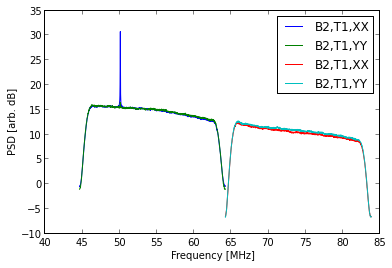# Basics¶

The LSL interface for DR spectrometer data is similar to that of DRX but there are a few key differences because they data have already been Fourier transformed and averaged in time.

First, download a snippet of DR spectrometer data:

In :
# This may take a bit...
import os
import urllib2
from lsl.reader import drx

if not os.path.exists('temp.drspec'):
fh1 = urllib2.urlopen('http://lda10g.alliance.unm.edu/tutorial/B0329+54/056770_000044687')
fh2 = open('temp.drspec', 'wb')
fh2.write(fh1.read(drx.FrameSize*300))
fh1.close()
fh2.close()

print "DR Spectrometer Size: %.1f kB" % (os.path.getsize('temp.drspec')/1024.)

DR Spectrometer Size: 1209.4 kB

The contents of the file can be found using a few of the top-level functions in the lsl.reader.drspec module:

In :
from lsl.reader import drspec

# Open the file
fh = open('temp.drspec', 'rb')

# What is the size in bytes of each frame?
print "Frame size:", drspec.getFrameSize(fh), "bytes"

# What is the sample rate?
print "Sample Rate:", drspec.getSampleRate(fh)/1e6, "MHz"

# How many frequency channels are in the data?
print "FFT length:", drspec.getTransformSize(fh), "channels"
print "-> %.3f kHz/channel" % (drspec.getSampleRate(fh)/1e3/drspec.getTransformSize(fh),)

# What type of data do we have?
print "Linear (XX, YY, etc.)?", drspec.containsLinearData(fh)
print "Stokes (I, V, etc.)?", drspec.containsStokesData(fh)

# What are the exact data products in the file?
print "Data products:", ", ".join(drspec.getDataProducts(fh))

# Close it out
fh.close()

Frame size: 16460 bytes
Sample Rate: 19.6 MHz
FFT length: 1024 channels
-> 19.141 kHz/channel
Linear (XX, YY, etc.)? True
Stokes (I, V, etc.)? False
Data products: XX, YY

To read in a single frame, use the modules read function:

In :
# Open the file and read in a frame
fh = open('temp.drspec', 'rb')
frame = drspec.readFrame(fh)

# Load in some basic information
beam = frame.parseID()
srate = frame.getSampleRate()
LFFT = drspec.getTransformSize(fh)
tInt = frame.header.nInts*LFFT/srate

# Print out some basic information
print "Beam:", beam
print "Timestamp:", frame.getTime()
print "Tuning 1:", frame.getCentralFreq(1)/1e6, "MHz"
print "Tuning 2:", frame.getCentralFreq(2)/1e6, "MHz"
print " "
print "Transform Length:", LFFT
print "Integration Time:", tInt, "s"

# Done
fh.close()

Beam: 2
Timestamp: 1398276221.03
Tuning 1: 54.4000009298 MHz
Tuning 2: 73.9999997616 MHz

Transform Length: 1024
Integration Time: 0.0401240816327 s

The time tags reported in the above example are in seconds since the UNIX epoch. These can be converted to Python datetime instances through:

In :
from datetime import datetime

# Open the file and read in a frame
fh = open('temp.drspec', 'rb')
frame = drspec.readFrame(fh)

# Load in some basic information
beam = frame.parseID()
srate = frame.getSampleRate()
LFFT = drspec.getTransformSize(fh)
tInt = frame.header.nInts*LFFT/srate

# Print out some basic information
print "Beam:", beam
print "Timestamp:", frame.getTime(), "(", datetime.utcfromtimestamp(frame.getTime()), ")"
print "Tuning 1:", frame.getCentralFreq(1)/1e6, "MHz"
print "Tuning 2:", frame.getCentralFreq(2)/1e6, "MHz"
print " "
print "Transform Length:", LFFT
print "Integration Time:", tInt, "s"

# Done
fh.close()

Beam: 2
Timestamp: 1398276221.03 ( 2014-04-23 18:03:41.034108 )
Tuning 1: 54.4000009298 MHz
Tuning 2: 73.9999997616 MHz

Transform Length: 1024
Integration Time: 0.0401240816327 s

The data product are stored as attributes to the frame.data object. You can either access these directly or through Python's getattr function:

In :
# Open the file and read in a frame
fh = open('temp.drspec', 'rb')
frame = drspec.readFrame(fh)

print "Tuning 1 XX mean:", frame.data.XX0.mean()
print "Tuning 2 XX mean:", frame.data.XX1.mean()
print " "

for tuning in (1, 2):
for prod in frame.getDataProducts():
data = getattr(frame.data, "%s%i" % (prod, tuning-1), None)
print "Tuning %i %s standard deviation:" % (tuning, prod), data.std()

# Done
fh.close()

Tuning 1 XX mean: 27.6883773804
Tuning 2 XX mean: 10.2878665924

Tuning 1 XX standard deviation: 10.0468772781
Tuning 1 YY standard deviation: 9.77003064685
Tuning 2 XX standard deviation: 3.85698524032
Tuning 2 YY standard deviation: 4.11942237842

# Plotting Spectra¶

Similar to DRX data you can also plot spectra from a DR spectrometer file. However, unlink DRX data, you do not need to make a call to SpecMaster since they data have already been transformed:

In :
%matplotlib inline
import numpy
from lsl.misc.mathutil import to_dB
from matplotlib import pyplot as plt

# Open the file
fh = open('temp.drspec', 'rb')

# Create the array that will hold the data
products = drspec.getDataProducts(fh)
nProd = len(products)*2
nChan = drspec.getTransformSize(fh)
data = numpy.zeros((nProd, 5, nChan), dtype=numpy.float32)

# Read in the first 5 frames
for i in xrange(5):
try:
frame = drspec.readFrame(fh)
except errors.syncError:
continue
except errors.eofError:
break

j = 0
for tuning in (1, 2):
for prod in products:
data[j,i,:] = getattr(frame.data, "%s%i" % (prod, tuning-1), None)
j += 1
fh.close()

# Integrate across the frames
spec = data.mean(axis=1)

# Compute the frequency bins
freq = numpy.fft.fftfreq(spec.shape, d=1.0/frame.getSampleRate())
freq = numpy.fft.fftshift(freq)
freq1 = freq + frame.getCentralFreq(1)
freq2 = freq + frame.getCentralFreq(2)

# Plot
beam = frame.parseID()

fig = plt.figure()
ax = fig.gca()
for i in xrange(spec.shape):
if i / len(products) == 0:
freq = freq1
else:
freq = freq2

ax.plot(freq/1e6, to_dB(spec[i,:]), label='B%i,T1,%s' % (beam, products[i % len(products)]))
ax.set_xlabel('Frequency [MHz]')
ax.set_ylabel('PSD [arb. dB]')
ax.legend(loc=0)
plt.show()

ERROR: Magic function matplotlib not found.